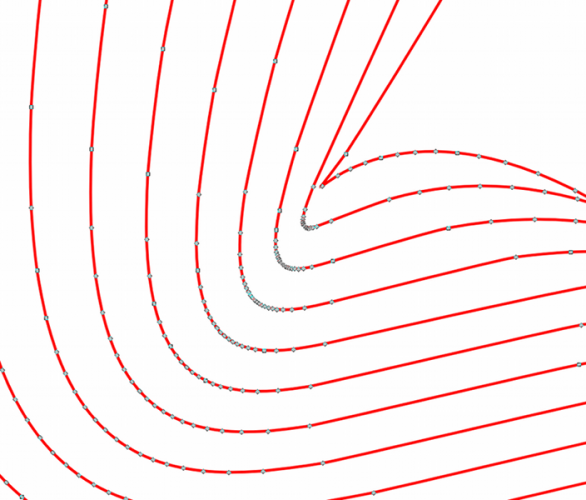Pretty Parametric Plots is an HTML5 application that I developed for fun (and practice) while preparing for software engineering interviews. The program was initially implemented in C++, but I ported it to JavaScript as a learning exercise. The program plots parametric equations of the following form:

$$!\displaystyle x(t) = R_1\cos(2\pi\omega_1t)e^{k_1t}+R_2\sin(2\pi\omega_2t+\phi e^{k_\phi t})e^{k_2 t}$$ $$!\displaystyle y(t) = R_1\sin(2\pi\omega_1t)e^{k_1t}+R_2\cos(2\pi\omega_2t+\phi e^{k_\phi t})e^{k_2 t}$$

as inline SVG images, using the relatively new <SVG> tag in HTML5.

#### Algorithm Summary

The algorithm is designed to quickly create very accurate, smooth curves, using a small number of data points. Each plot is defined using a cubic Bézier spline. Rather than spacing the component curves equally in the parameter, $$t$$, the algorithm adaptively computes the interval, $$\delta t$$, because regions of high curvature require smaller intervals than regions of low curvature. This is illustrated in the image below.The above image shows a zoomed-in plot with quite stringent quality settings. The gray points indicate nodes (the endpoints of the individual Bézier curves within the spline). Note that sharp turns are handled efficiently by simply limiting the distance between nodes; the shortest allowed interval is approximately one third of a pixel width on the host computer’s display device. This also prevents the application from wasting time on sub-pixel structures, such as tiny loops. With additional effort, the total number of points could greatly be reduced, while retaining accuracy. Since this optimization also significantly slows the plotting operation, the application does not take this extra step.

#### Examples

The current version of the application is rather simple. The user can specify the constants that define a particular plot and then adjust the width and color of the line, as well as the color of the background. The following features may eventually be added: